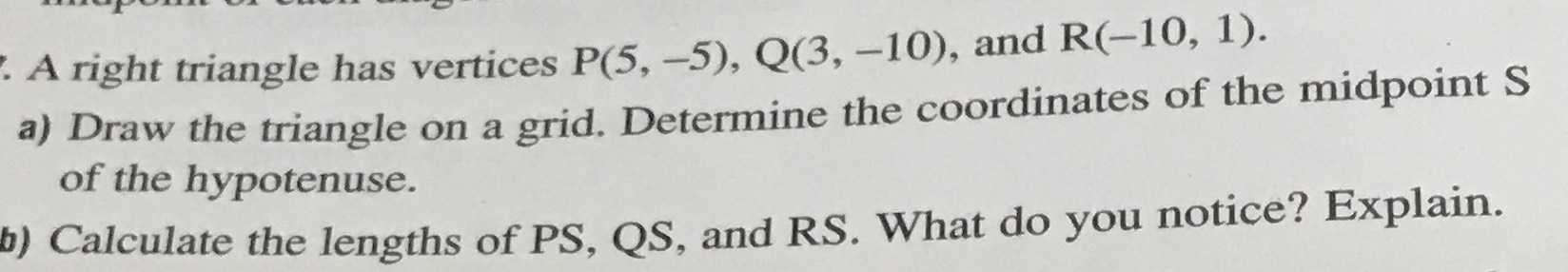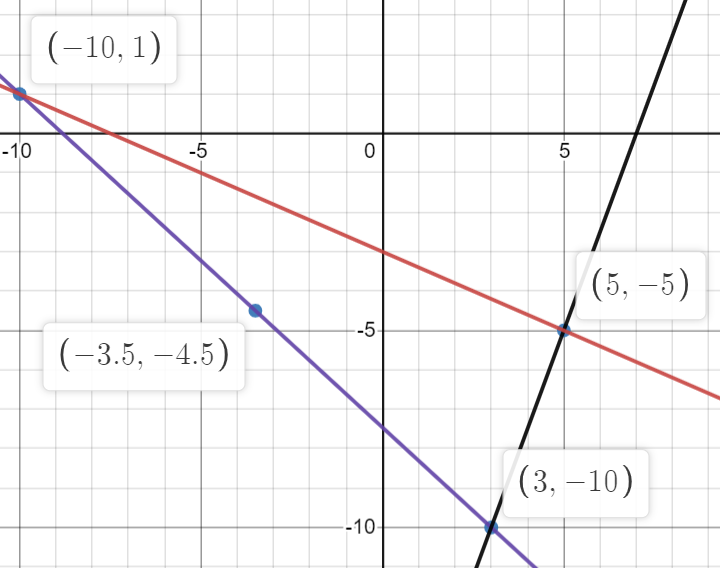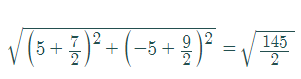### Still have math questions?

Trigonometry
QuestionA right triangle has vertices $$P ( 5 , - 5 ) , Q ( 3 , - 10 ) ,$$ and $$R ( - 10,1 ) .$$

a) Draw the triangle on a grid. Determine the coordinates of the midpoint $$S$$ of the hypotenuse. b) Calculate the lengths of PS, QS, and RS. What do you notice? Explain.

a.b.PS=